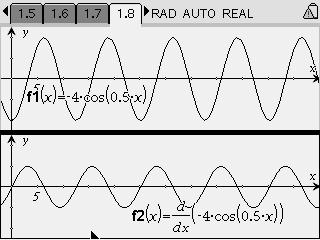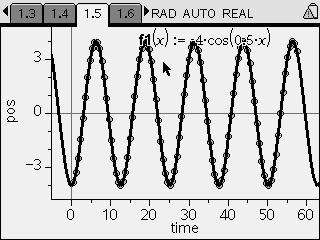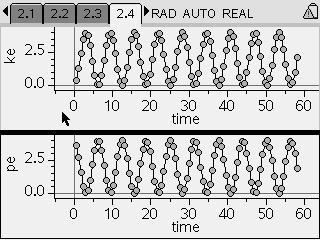# Activities

••• ##### Subject Area

• Science: Physics: Dynamics
• Science: Physics: Work, Energy and Momentum

• ##### Author9-12

45 Minutes

• ##### Device
• TI-Nspire™
• TI-Nspire™ CAS
• ##### Software

TI-Nspire™
TI-Nspire™ CAS

1.7

• ##### Other Materials
• pen or pencil
• blank sheet of paper
• ##### Report an Issue

Energy of Free Oscillations#### Activity Overview

Students explore energy transformation and energy conservation in simulated spring-mass oscillations.

#### Key Steps

•Students use a simulated spring-mass system to investigate position, velocity, and conservation of mechanical energy in a simple harmonic oscillator.

First, students use the simulation to collect data on the position of the mass over time as the spring oscillates. They study the graph and determine the equation that describes the position of the mass.

•Next, students take the derivative of the position function to find the equation describing velocity as a function of time. They plot both functions to investigate the relationship between position and velocity for a simple harmonic oscillator.

•Finally, students use another simulation to record the position and velocity of the mass over time. They use this information to calculate kinetic energy and potential energy as a function of time. By comparing the two graphs, students confirm that mechanical energy is conserved in the system.